Custom SearchTRIAC The TRIAC is a three-terminal device similar in construction and operation to the SCR. The TRIAC controls and conducts current flow during both alternations of an ac cycle, instead of only one. The schematic symbols for the SCR and the TRIAC are compared in figure 3-23. Both the SCR and the TRIAC have a gate lead. However, in the TRIAC the lead on the same side as the gate is "main terminal 1," and the lead opposite the gate is "main terminal 2." This method of lead labeling is necessary because the TRIAC is essentially two SCRs back to back, with a common gate and common terminals. Each terminal is, in effect, the anode of one SCR and the cathode of another, and either terminal can receive an input. In fact, the functions of a TRIAC can be duplicated by connecting two actual SCRs as shown in figure 3-24. The result is a three-terminal device identical to the TRIAC. The common anode-cathode connections form main terminals 1 and 2, and the common gate forms terminal 3. Figure 3-23. - Comparison of SCR and TRIAC symbols.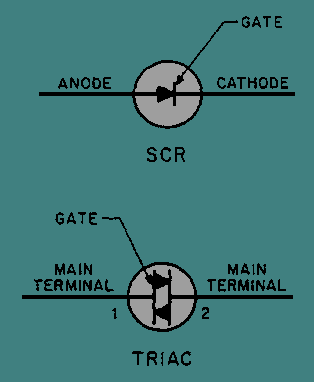Figure 3-24. - Back to back SCR equivalent circuit.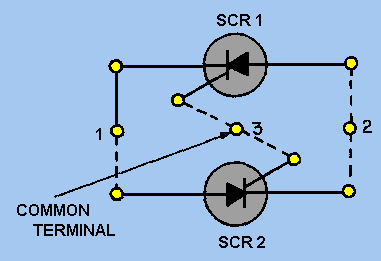The difference in current control between the SCR and the TRIAC can be seen by comparing their operation in the basic circuit shown in figure 3-25. In the circuit shown in view A, the SCR is connected in the familiar half-wave arrangement. Current will flow through the load resistor (RL) for one alternation of each input cycle. Diode CR1 is necessary to ensure a positive trigger voltage. Figure 3-25A. - Comparison of SCR and TRIAC circuits.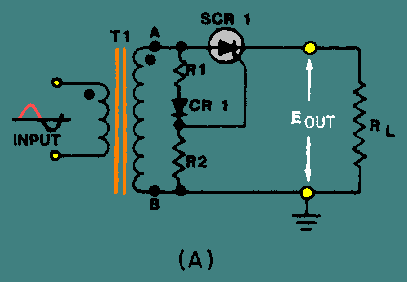In the circuit shown in view B, with the TRIAC inserted in the place of the SCR, current flows through the load resistor during both alternations of the input cycle. Because either alternation will trigger the gate of the TRIAC, CR1 is not required in the circuit. Current flowing through the load will reverse direction for half of each input cycle. To clarify this difference, a comparison of the waveforms seen at the input, gate, and output points of the two devices is shown in figure 3-26. Figure 3-25B. - Comparison of SCR and TRIAC circuits.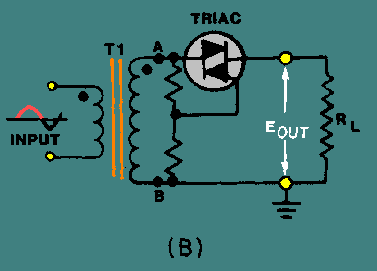Figure 3-26. - Comparison of SCR and TRIAC waveforms.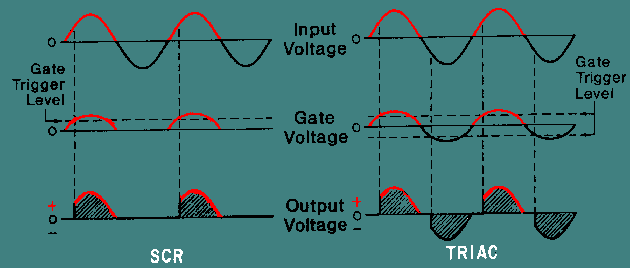Q.17 The TRIAC is similar in operation to what device?Q.18 When used for ac current control, during which alternation of the ac cycle does the TRIAC control current flow?Privacy Statement - Copyright Information. - Contact Us